Class VI - Mathematics

# NCERT Solutions: Chapter - 4 Basic Geometrical Ideas

Other Chapters

Page 74

Exercise 4.1

Q1. Use the figure to name :
(a) Five points
(b) A line
(c) Four rays
(d) Five line segments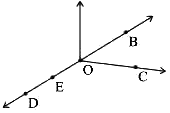Answer:

(a) Five points are D, E, O, B and C.
(b) $\overline{\mathrm{DB}}$
(c)
(d)

Q2. Name the line given in all possible (twelve) ways, choosing only two letters at a time from the four given.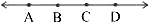Answer

Q3. Use the figure to name :
(a) Line containing point E.
(b) Line passing through A.
(c) Line on which O lies
(d) Two pairs of intersecting lines.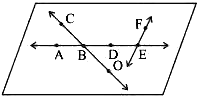Answer:

(a) $\overline{\mathrm{AE}}$ and $\overline{\mathrm{FE}}$
(b) $\overline{\mathrm{AE}}$
(c) $\overline{\mathrm{CO}}$
(d) $\overline{\mathrm{CO}}$ & $\overline{\mathrm{AE}}$, $\overline{\mathrm{FE}}$ & $\overline{\mathrm{AE}}$

Q4. How many lines can pass through (a) one given point? (b) two given points?

(a) Infinite lines can pass through one given point.
(b) Only one line can pass through two given points.

Q5. Draw a rough figure and label suitably in each of the following cases:
(a) Point P lies on AB.
(b) $\overline{\mathrm{XY}}$ and $\overline{\mathrm{PQ}}$ intersect at M.
(c) Line l contains E and F but not D.
(d) $\overline{\mathrm{OP}}$ and $\overline{\mathrm{OQ}}$ meet at O.

(a)(b)(c)(d)Q6. Consider the following figure of line $\overline{\mathrm{MN}}$. Say whether following statements are true or false in context of the given figure.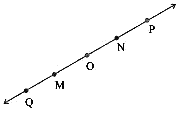(a) Q, M, O, N, P are points on the line $\overline{\mathrm{MN}}$.
(b) M, O, N are points on a line segment $\overline{\mathrm{MN}}$.
(c) M and N are end points of line segment $\overline{\mathrm{MN}}$.
(d) O and N are end points of line segment $\overline{\mathrm{OP}}$.
(e) M is one of the end points of line segment $\overline{\mathrm{QO}}$.
(f) M is point on ray $\stackrel{\to }{\mathrm{OP}}$.
(g) Ray $\stackrel{\to }{\mathrm{OP}}$ is different from ray $\stackrel{\to }{\mathrm{QP}}$.
(h) Ray $\stackrel{\to }{\mathrm{OP}}$ is same as ray $\stackrel{\to }{\mathrm{OM}}$.
(i) Ray $\stackrel{\to }{\mathrm{OM}}$ is not opposite to ray $\stackrel{\to }{\mathrm{OP}}$.
(j) O is not an initial point of $\stackrel{\to }{\mathrm{OP}}$.
(k) N is the initial point of $\overline{\mathrm{NP}}$ and $\overline{\mathrm{NM}}$.

(a) True, point Q and P are on the line $\overline{\mathrm{MN}}$. A line through two points when extends indefinitely in both directions, can contains a countless number of points.

(b) True, M, O, N are points on a line segment $\overline{\mathrm{MN}}$.

(c) True, M and N are end points of line segment $\overline{\mathrm{OP}}$.

(d) False, the end points of line segment $\overline{\mathrm{MN}}$ are O and P and not O and N.

(e) False, M is not one of the end points of line segment $\overline{\mathrm{QO}}$ but is a point in line $\overline{\mathrm{QO}}$.

(f) False, point M is outside ray $\stackrel{\to }{\mathrm{OP}}$.

(g) True, Ray $\stackrel{\to }{\mathrm{OP}}$ is different from ray $\stackrel{\to }{\mathrm{QP}}$.

(h) False, Ray $\stackrel{\to }{\mathrm{OP}}$ is opposite to ray $\stackrel{\to }{\mathrm{OM}}$.

(i) False, Ray $\stackrel{\to }{\mathrm{OM}}$ is opposite to ray $\stackrel{\to }{\mathrm{OP}}$.

(j) False, O is the initial point of $\stackrel{\to }{\mathrm{OP}}$.

(k) True, N is the initial point of $\overline{\mathrm{NP}}$ and $\overline{\mathrm{NM}}$.

Page 78

Exercise 4.2

Q1. Classify the following curves as (i) Open or (ii) Closed.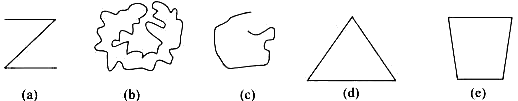Answer:

(a) Open
(b) Closed
(c) Open
(d) Closed
(e) Closed

Q2. Draw rough diagrams to illustrate the following :
(a) Open curve
(b) Closed curve.

(a)(b)Q3. Draw any polygon and shade its interior.

A figure is a polygon if it is a simple closed figure made up entirely of line segments.Q4. Consider the given figure and answer the questions: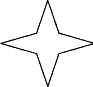(a) Is it a curve?
(b) Is it closed?

(a) Yes, it is a curve.
(b) Yes, it is closed.

Q5. Illustrate, if possible, each one of the following with a rough diagram:
(a) A closed curve that is not a polygon.
(b) An open curve made up entirely of line segments.
(c) A polygon with two sides.

(a)(b)(c) A figure is a polygon if it is a simple closed figure made up entirely of line segments. Hence a polygon with two sides is not possible.

Page 80

Exercise 4.3

Q1. Name the angles in the given figure.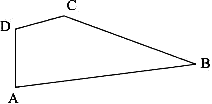∠DAB, ∠CBA, ∠CDA, ∠BCD

Q2. In the given diagram, name the point(s)
(a) In the interior of
DOE
(b) In the exterior of
EOF
(c) On
EOF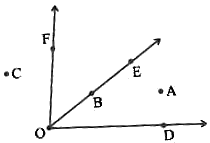(a) Point in the interior of ∠DOE is A.
(b) Points in the exterior of ∠EOF are C, A and D.
(c) Points on ∠EOF are E, B, O and F.

Q3. Draw rough diagrams of two angles such that they have
(a) One point in common.
(b) Two points in common.
(c) Three points in common.
(d) Four points in common.
(e) One ray in common.

(a)(b)(c)(d)(e)Page 81

Exercise 4.4

Draw a rough sketch of a triangle ABC. Mark a point P in its interior and a point Q in its exterior. Is the point A in its exterior or in its interior?Point A lies on the triangle ABC.

Q2. (a) Identify three triangles in the figure.
(b) Write the names of seven angles.
(c) Write the names of six line segments.
(d) Which two triangles have
B as common?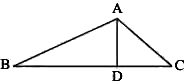(a) Three triangles in the given figure are ∆ABD, ∆ADC and ∆ABC.
(b) The seven angles in the given figure are ∠ABD, ∠BDA, ∠DAB, ∠ADC, ∠DCA, ∠CAD and ∠CAB.
(c) The six line segments in the given figure are $\overline{\mathrm{AB}}$, $\overline{\mathrm{AC}}$, $\overline{\mathrm{AD}}$, $\overline{\mathrm{DC}}$, $\overline{\mathrm{BD}}$ and $\overline{\mathrm{BC}}$.

(d) The two triangles that have ∠B as common are ∆ABD and ∆ABC.

Page 82

Exercise 4.5

Q1. Draw a rough sketch of a quadrilateral PQRS. Draw its diagonals. Name them. Is the meeting point of the diagonals in the interior or exterior of the quadrilateral?Q2. Draw a rough sketch of a quadrilateral KLMN. State,
(a) two pairs of opposite sides,
(b) two pairs of opposite angles,
(c) two pairs of adjacent sides,
(d) two pairs of adjacent angles.Q3. Investigate : Use strips and fasteners to make a triangle and a quadrilateral. Try to push inward at any one vertex of the triangle. Do the same to the quadrilateral.
Is the triangle distorted? Is the quadrilateral distorted? Is the triangle rigid? Why is it that structures like electric towers make use of triangular shapes and not quadrilaterals?

On pushing inward at any one vertex of the triangle we observe that the triangle is not distorted.
When we push inward any one vertex of the qadrilateral we observe that it gets distorted.
Yes, the triangle is rigid.
Since the triangle is rigid and does not distort, structures like electric towers make use of triangular shapes and not quadrilaterals.

Page 84

Exercise 4.6

From the figure, identify :
(a) the centre of circle
(c) a diameter
(d) a chord
(e) two points in the interior
(f) a point in the exterior
(g) a sector
(h) a segment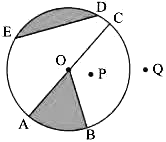(a) The centre of circle is point O.
(b) Three radii are $\overline{\mathrm{OA}}$, $\overline{\mathrm{OB}}$ and $\overline{\mathrm{OC}}$.
(c) The diameter is $\overline{\mathrm{AC}}$.
(d) A chord is $\overline{\mathrm{ED}}$.
(e) Two points in the interior are P and O.
(f) A point in the exterior is Q.
(g) A sector is shaded area AOB.
(h) A segment is shaded area ED.

Q2. (a) Is every diameter of a circle also a chord?
(b) Is every chord of a circle also a diameter?

(a) Yes, every diameter of a circle is also a chord. A diameter is a chord passing through the centre of the circle.
(b) No, every chord of a circle is not also a diameter. A chord of a circle is a line segment joining any two points on the circle. It may or may not pass through the centre of the circle.

Q3. Draw any circle and mark
(a) its centre
(c) a diameter
(d) a sector
(e) a segment
(f) a point in its interior
(g) a point in its exterior
(h) an arc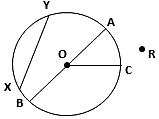(a) its centre = O
(b) a radius = $\overline{\mathrm{OA}}$
(c) a diameter = $\overline{\mathrm{AB}}$
(d) a sector = AOC
(e) a segment = XY
(f) a point in its interior = O
(g) a point in its exterior = R
(h) an arc = AC

Q4. Say true or false :
(a) Two diameters of a circle will necessarily intersect.
(b) The centre of a circle is always in its interior.